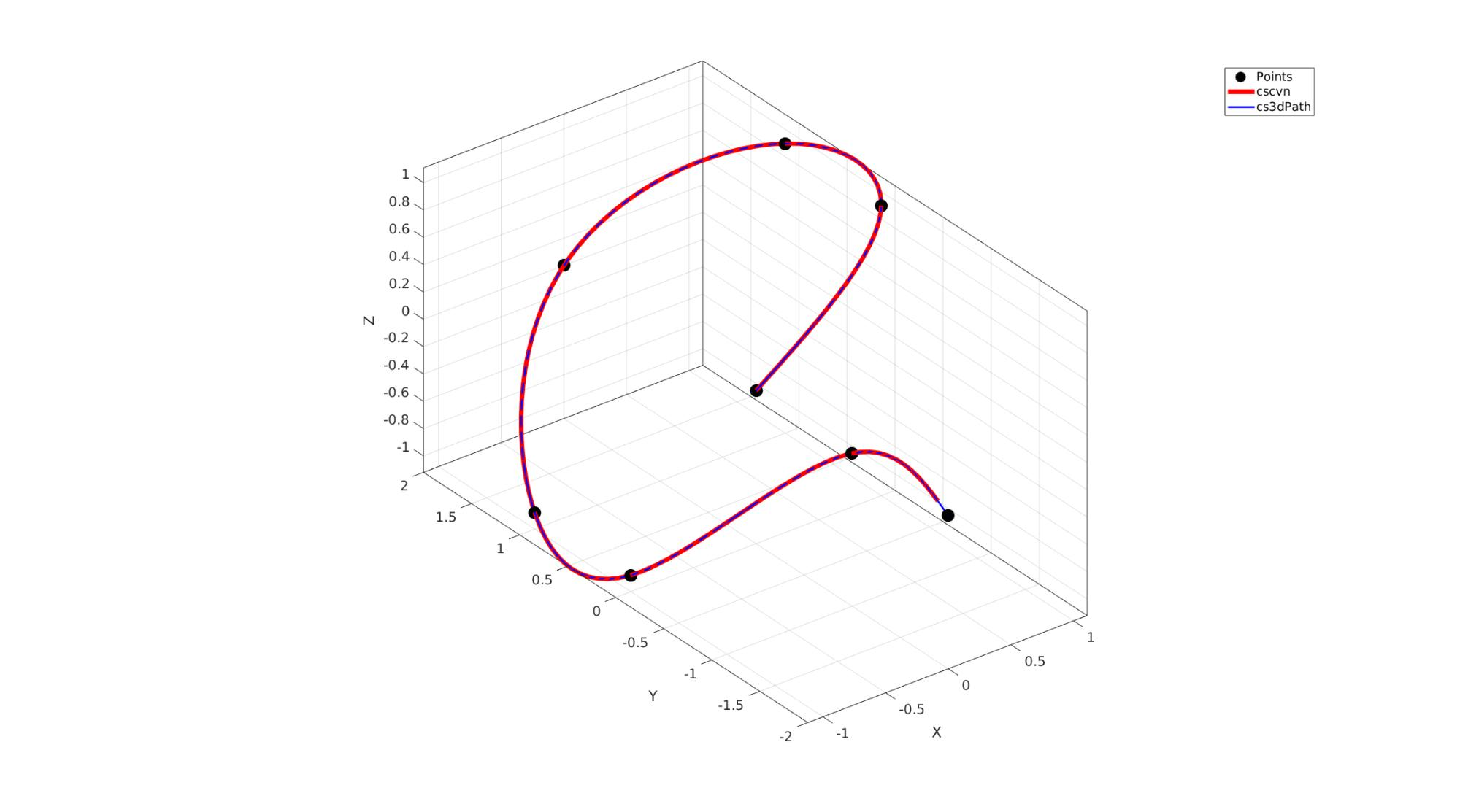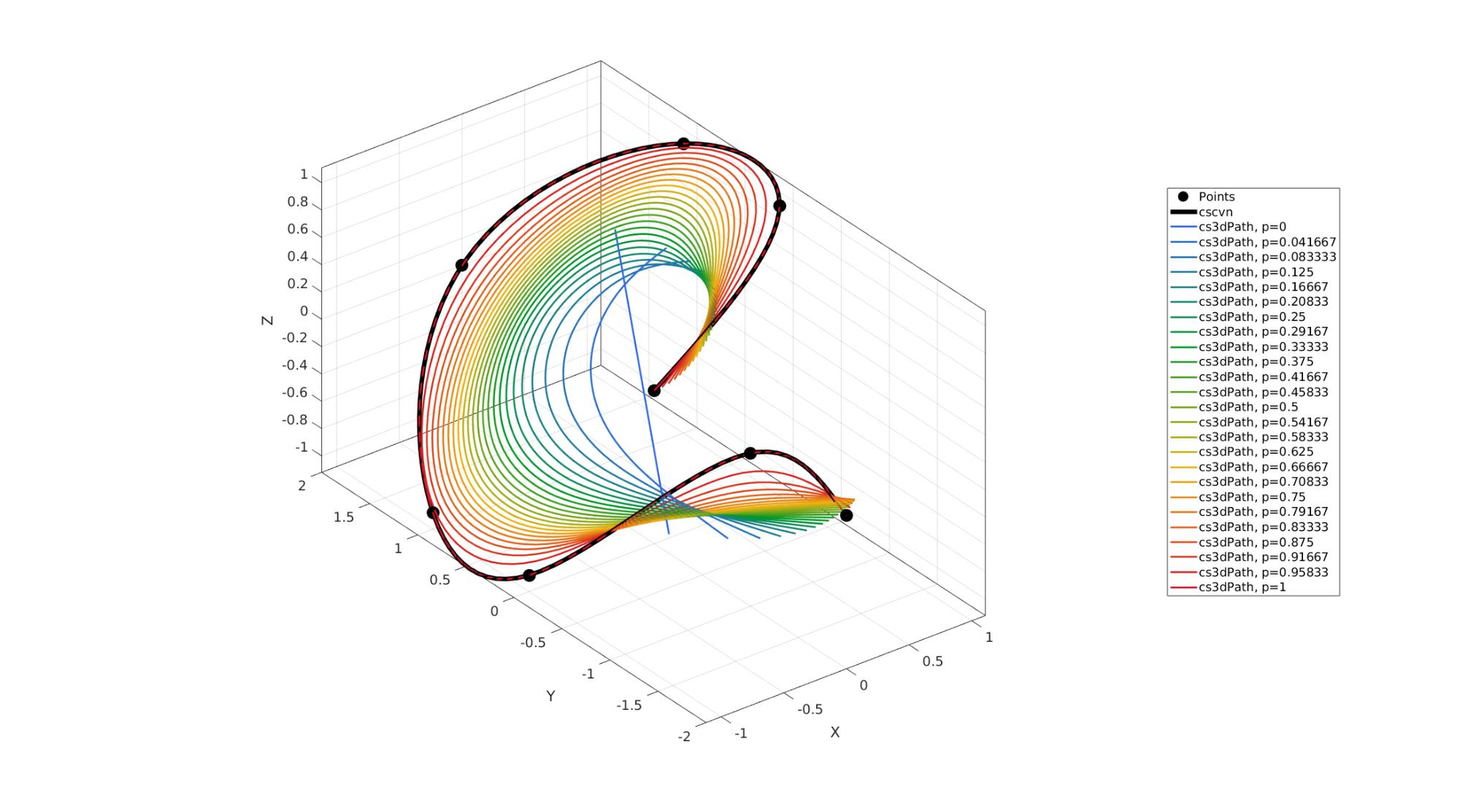# cs3dPath

Below is a demonstration of the features of the cs3dPath function

## Contents

```clear; close all; clc;
```

## Syntax

[pp,t]=cs3dPath(V,p,w);

## Description

This function generates the pp-form for a cubic-smoothing spline to the 2D or 3D curve defined by V.

Equivalent to CSCVN performance if p=1 and w=ones(size(V,1),1)

## Examples

Plot settings

```markerSize=50;
lineWidth1=5;
lineWidth2=2;
fontSize=15;
```

## Example

```V = [0,0;1,0;1,1;0,2;-1,1;-1,0;0,-1;0,-2];
V(:,3) = V(:,1);

f1=cscvn(V');
V1 = fnval(f1,linspace(0,size(V,1),100))';

p=1;
w=ones(size(V,1),1);

[pp,t]=cs3dPath(V,p,w);
T=linspace(0,max(t),100);
V2=ppval(pp,T)';

cFigure; hold on;
hp1=plotV(V,'k.','MarkerSize',markerSize);
hp2=plotV(V1,'r-','LineWidth',lineWidth1);
hp3=plotV(V2,'b-','LineWidth',lineWidth2);
legend([hp1 hp2 hp3],{'Points','cscvn','cs3dPath'});
axisGeom;
set(gca,'FontSize',fontSize);
drawnow;
``````cFigure; hold on;
hp1=plotV(V,'k.','MarkerSize',markerSize);
hp2=plotV(V1,'k-','LineWidth',lineWidth1);

% legend([hp1 hp2 hp3],{'Points','cscvn','cs3dPath'});
axisGeom;
set(gca,'FontSize',fontSize);

hp=[hp1 hp2];
legendText={'Points','cscvn'};
P=linspace(0,1,25);
c=gjet(numel(P));
for q=1:1:numel(P)
p=P(q);
[pp,t]=cs3dPath(V,p,w);
T=linspace(0,max(t),100);
V2=ppval(pp,T)';
hp(end+1)=plotV(V2,'b-','LineWidth',lineWidth2,'Color',c(q,:));
legendText{end+1}=['cs3dPath, p=',num2str(p)];
end

legend(hp,legendText,'Location','EastOutside');
drawnow;
```GIBBON www.gibboncode.org

Kevin Mattheus Moerman, [email protected]

GIBBON footer text

GIBBON: The Geometry and Image-based Bioengineering add-On. A toolbox for image segmentation, image-based modeling, meshing, and finite element analysis.

Copyright (C) 2006-2023 Kevin Mattheus Moerman and the GIBBON contributors

This program is free software: you can redistribute it and/or modify it under the terms of the GNU General Public License as published by the Free Software Foundation, either version 3 of the License, or (at your option) any later version.

This program is distributed in the hope that it will be useful, but WITHOUT ANY WARRANTY; without even the implied warranty of MERCHANTABILITY or FITNESS FOR A PARTICULAR PURPOSE. See the GNU General Public License for more details.

You should have received a copy of the GNU General Public License along with this program. If not, see http://www.gnu.org/licenses/.